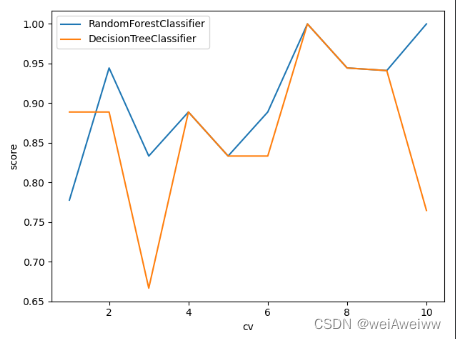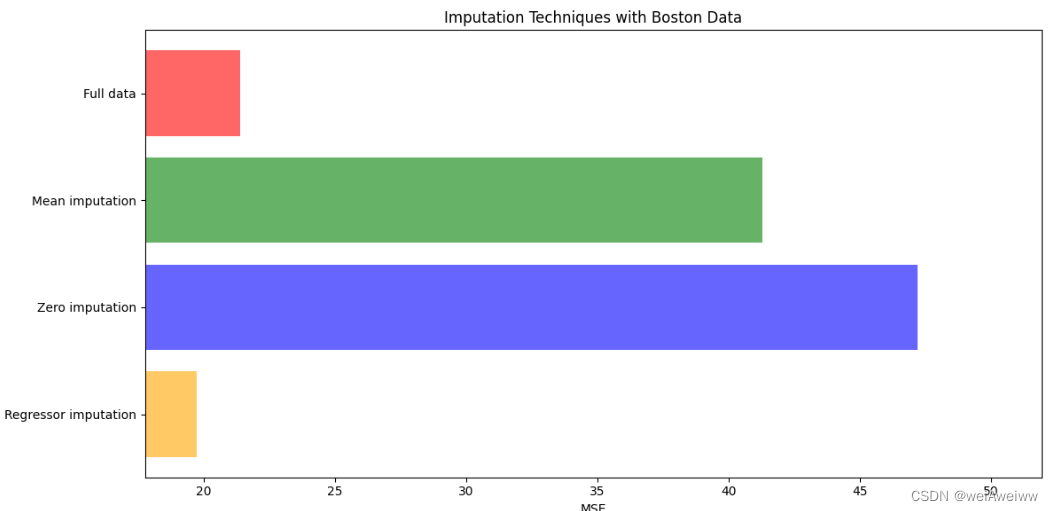• 随机森林分类器python
2022-07-29 20:01:11

### Python:实现random forest classifier随机森林分类器算法

# Random Forest Classifier Example
from matplotlib import pyplot as plt
from sklearn.datasets import load_iris
from sklearn.ensemble import RandomForestClassifier
from sklearn.metrics import plot_confusion_matrix
from sklearn.model_selection import train_test_splitpython 随机森林
更多相关内容
•python 算法
• python编写的随机森林分类器。 用法 from sklearn . datasets import load_digits from sklearn import cross_validation import numpy as np from randomforest import RandomForestClassifier digits = load_...Python
• 【项目实战】基于Python实现随机森林分类模型(RandomForestClassifier)项目 资料说明：包括数据集+源代码+Pdf文档说明。 资料内容包括： 1)项目背景； 2)获取数据； 3)数据预处理： (1)导入程序库并读取数据 ...
• 随机森林分类器用相同的训练数据同时搭建多个独立的分裂模型，然后通过投票的方式，以少数服从多数的原则作出最终分类的决策。在相同的训练数据上同时搭建多棵决策树，每棵决策树会放弃固定的排序算法，随机选取特征...
• 机器学习算法（8）python实现随机森林分类） 可以把随机森林看成是决策树的集 合。随机森林背后的逻辑是对分别受较大方差影响的多个决策树取平均值， 以建立一个具有更好的泛化性能和不易过拟合的强大模型。 ...

# python实现随机森林（分类）

可以把随机森林看成是决策树的集 合。随机森林背后的逻辑是对分别受较大方差影响的多个决策树取平均值， 以建立一个具有更好的泛化性能和不易过拟合的强大模型。

import matplotlib.pyplot as plt
import numpy as np
from matplotlib.colors import ListedColormap
from sklearn import datasets
from sklearn.model_selection import train_test_split
from sklearn.ensemble import RandomForestClassifier

X = iris.data[:, [2, 3]]
y = iris.target
print('Class labels:', np.unique(y))

# Splitting data into 70% training and 30% test data:
X_train, X_test, y_train, y_test = train_test_split(
X, y, test_size=0.3, random_state=1, stratify=y)
X_combined = np.vstack((X_train, X_test))
y_combined = np.hstack((y_train, y_test))

def plot_decision_regions(X, y, classifier, test_idx=None, resolution=0.02):
# setup marker generator and color map
markers = ('s', 'x', 'o', '^', 'v')
colors = ('red', 'blue', 'lightgreen', 'gray', 'cyan')
cmap = ListedColormap(colors[:len(np.unique(y))])

# plot the decision surface
x1_min, x1_max = X[:, 0].min() - 1, X[:, 0].max() + 1
x2_min, x2_max = X[:, 1].min() - 1, X[:, 1].max() + 1
xx1, xx2 = np.meshgrid(np.arange(x1_min, x1_max, resolution),
np.arange(x2_min, x2_max, resolution))
Z = classifier.predict(np.array([xx1.ravel(), xx2.ravel()]).T)
Z = Z.reshape(xx1.shape)
plt.contourf(xx1, xx2, Z, alpha=0.3, cmap=cmap)
plt.xlim(xx1.min(), xx1.max())
plt.ylim(xx2.min(), xx2.max())

for idx, cl in enumerate(np.unique(y)):
plt.scatter(x=X[y == cl, 0],
y=X[y == cl, 1],
alpha=0.8,
c=colors[idx],
marker=markers[idx],
label=cl,
edgecolor='black')

# highlight test samples
if test_idx:
# plot all samples
X_test, y_test = X[test_idx, :], y[test_idx]

plt.scatter(X_test[:, 0],
X_test[:, 1],
c='y',
edgecolor='black',
alpha=1.0,
linewidth=1,
marker='o',
s=100,
label='test set')

forest = RandomForestClassifier(criterion='gini',
n_estimators=25,
random_state=1,
n_jobs=2)
forest.fit(X_train, y_train)

plot_decision_regions(X_combined, y_combined,
classifier=forest, test_idx=range(105, 150))

plt.xlabel('petal length [cm]')
plt.ylabel('petal width [cm]')
plt.legend(loc='upper left')
plt.tight_layout()
#plt.savefig('images/03_22.png', dpi=300)
plt.show()



运行结果：
Class labels: [0 1 2]

运行结果图：展开全文python 机器学习 决策树
• ## Python随机森林分类

千次阅读 2021-03-15 20:43:50
Python 随机森林分类 1 声明 本文的数据来自网络，部分代码也有所参照，这里做了注释和延伸，旨在技术交流，如有冒犯之处请联系博主及时处理。 2 决策树分类简介 相关概念见下： 决策树的最大问题是树在训练集...

# 1 声明

本文的数据来自网络，部分代码也有所参照，这里做了注释和延伸，旨在技术交流，如有冒犯之处请联系博主及时处理。

# 2 决策树分类简介

相关概念见下：

决策树的最大问题是树在训练集生成的过于茂密，导致过拟合(即在训练集上表现卓越但在测试集上效果很差)。这时随机森林就应运而生了。在随机森林里会有很多决策树，而每颗决策树只接收自举样本且每个节点仅围绕部分特征寻找最好的分割。随机决策树的森林最后通过投票的方式选出最后的预测分类。

注：自举样本是指从一个较大的样本中 "自举 "出来的较小样本。Bootstrap是一种重新取样的方法，即从一个原始样本中反复抽取大量相同大小的小样本，并进行替换。

随机森林分类里的几个参数：

max_features：每个节点选择的特征的最大数

boostrap：指定是否以自举采样

n_estimators： 指定决策树的个数

n_jobs：并行数量，等于-1是最大化计算资源

# 3 随机森林分类代码与注释示例

# 加载随机森林分类包
from sklearn.ensemble import RandomForestClassifier
from sklearn import datasets
# 加载数据与模型创建
features = iris.data
target = iris.target
randomforest = RandomForestClassifier(random_state=0, n_jobs=-1)
# 训练模型
model = randomforest.fit(features, target)
# 预测分类
observation = [[ 5, 4, 3, 2]]
# Predict observation's class
print(model.predict(observation))

# 4 总结

展开全文• 随机森林是数据挖掘中非常常用的分类预测算法，以分类或回归的决策树为基分类器。算法的一些基本要点： *对大小为m的数据集进行样本量同样为m的有放回抽样； *对K个特征进行随机抽样，形成特征的子集，样本量的确定...
• 在前面的内容中，已经对决策树解决分类回归问题分别做了阐述，今天走进随机森林的世界。什么是森林呢，对，好多树在一起我们就叫它森林，为什么是随机呢，因为这片森林可能不一样，这就涉及到参数的设定了（就像之前...

目录

集成算法

sklearn中的随机森林

随机森林分类器

随机性参数

属性

案例代码

随机森林回归器

案例代码

在前面的内容中，已经对决策树解决分类回归问题分别做了阐述，今天走进随机森林的世界。

什么是森林呢，对，好多树在一起我们就叫它森林，为什么是随机呢，因为这片森林可能不一样，这就涉及到参数的设定了（就像之前决策树的参数一样）。

名字的意义明白了，那这最终的结果是怎么得出来的呢？其实很简单，我们知道一棵决策树会给出一个结果，多棵树就会给出多个结果，最后”投票“表决，得票高的就是最终随机森林给出的结果喽。（当然，这里的解释较为简单，想知道具体原理可以阅读《数据挖掘导论》和西瓜书。）

# 集成算法

集成学习算法本身不算一种单独的机器学习算法,而是通过构建并结合多个机器学习器来完成学习任务。

目标：集成算法会考虑多个评估器的建模结果，汇总之后得到一个综合的结果，以此获取比单个模型更好的回归或者分类效果。

集成评估器：多个模型集成后的模型，如随机森林。

分类：bagging（袋装法），boosting（提升法），stacking。主要介绍前两个。

bagging（袋装法）：模型之间相互独立，互相平行，如随机森林。# sklearn中的随机森林

## 随机森林分类器

参数列表几乎与决策树的相同，重要的有：

criterion,      max_depth,     min_samples_leaf,     min_samples_split,    max_features,   min_impurity_decrease

n_estimators树木的数量，越大往往越好，但达到一定程度之后精确性往往会上下波动，默认10，0.22版本会改为100，一般0-200选一个比较好。

.除此之外，还需要注意的是关于随机性的这两个参数：

### 随机性参数

1、random_state

random_state固定时，随机森林中生成的是一组固定的树，但每棵树依然不一致，这是随机挑选特征进行分枝的方法。当随机性越大的时候，袋装法的效果一般会越来越好，用袋装法集成时，基分类器应该是相互独立，是不相同的。

但是局限性强，数据可能没有足够多的特征构建成千上万棵不同的树，因此还需要其他方式。

2、bootstrap & oob_score

使用不同的训练集，袋装法有放回随机抽样技术来形成不同的训练数据，bootstrap就是控制抽样技术的参数。

但是这又会引出如下问题：一些样本可能在同一个自助集出现多次，而其他一些却可能被忽略。一般来说，自助集大约包含63%的原始数据，计算方式：1-（1-1/n)**n，这里是按逆向思维进行计算的：每次都不被选中，总共进行n次，总概率1减去该值即为如上结果。

那既然大约63%的数据被使用，就会有约37%的数据未被使用，这个数据集叫做袋外数据（out of bag data,oob除开始划分的测试集，这些也可以用来当测试集——所以，在使用随机森林时，可以不划分测试集和训练集，只需要袋外数据来测试我们的模型即可，当然也不绝对，因为当n和n_estimators都不够大时，很可能就没有数据掉到袋外，自然也就无法使用oob数据来测试模型。

### 属性

相较决策树，随机森林除feature_importances属性外，

#重要性
importance = clf.feature_importances_
feature_importance = [(feature,importance.round(3)) for feature,importance in zip(feature_name,importance)]
#排序
feature_importance = sorted(feature_importance,key=lambda x :x,reverse=True)
#对应进行打印
[print('variable:{:20} importance: {}'.format(*pair)) for pair in feature_importance]

还有如下两个属性：

1、estimators_

#随机森林的重要属性：estimators_,查看森林中树的情况

print(rf.estimators_)

rf.estimators_.random_state

2、 oob_score

rf = RandomForestClassifier(n_estimators=25,random_state=42,oob_score=True)
oob = rf.oob_score_

### 案例代码

from sklearn.ensemble import RandomForestClassifier
from sklearn.tree import DecisionTreeClassifier
import matplotlib.pyplot as plt
from sklearn.datasets import load_wine
from sklearn.model_selection import train_test_split
from sklearn.model_selection import cross_val_score

wine = load_wine() #字典
print(wine.data) #可以通过键取值
print(wine.data.shape)
print(wine.target)

xtrain,xtest,ytrain,ytest = train_test_split(wine.data,wine.target,test_size=0.3)
rf = RandomForestClassifier(n_estimators=25,random_state=42,oob_score=True)
clf = DecisionTreeClassifier(random_state=42)
#交叉验证
cross_rf = cross_val_score(rf,wine.data,wine.target,cv=5)
cross_clf = cross_val_score(clf,wine.data,wine.target,cv=5)

rf.fit(xtrain,ytrain)
score_rf = rf.score(xtest,ytest)
oob = rf.oob_score_
clf.fit(xtrain,ytrain)
score_clf = clf.score(xtest,ytest)

print('===',score_clf.round(3),score_rf.round(3),oob.round(3))
plt.plot(range(1,6),cross_clf,label='DecisionTreeClassifier')
plt.plot(range(1,6),cross_rf,label='RandomForestClassifier')
plt.xlabel('cv')
plt.ylabel('score')
plt.legend()
plt.show()

#随机森林的重要属性：estimators_,查看森林中树的情况
print(rf.estimators_)
for i in range(len(rf.estimators_)):
print(rf.estimators_[i].random_state)

################换一种写法##############
cv = 10
for model in [RandomForestClassifier(n_estimators=5,random_state=42),DecisionTreeClassifier(random_state=42)]:
cross = cross_val_score(model, wine.data, wine.target, cv=cv)
plt.plot(range(1,cv+1),cross,label='{}'.format(str(model).split('(')))
plt.xlabel('cv')
plt.ylabel('score')
plt.legend()
plt.show()

##################学习曲线################
# superpa = []
# for i in range(200):
#     rf = RandomForestClassifier(random_state=42,n_estimators=i+1)
#     sc = cross_val_score(rf,wine.data,wine.target,cv=10).mean()
#     superpa.append(sc)
# plt.plot(range(1,201),superpa)
# print(max(superpa),superpa.index((max(superpa))))
# plt.show()

import numpy as np
from scipy.special import  comb
# 建立25棵树，当且仅当有13棵以上的树判断错误时，随机森林才会报错
# 如果一棵树的准确率在0.85上下浮动，那20棵树以上判断错误的概率
sum = np.array([comb(25,i)*(0.2**i)*((1-0.2)**(25-i)) for i in range(13,26)]).sum()
print(sum)## 随机森林回归器

测试集与训练集的划分思想：

xtrain：特征不缺失的值对应其他的n-1个特征 + 本来的标签

ytrain：特征不缺失的值

xtest：特征缺失的值对应其他的n-1个特征 + 本来的标签

ytest:特征缺失的值

适用于某一个特征大量缺失，而其他特征相对完整。

对，相对完整，也就是其他特征也可能存在缺失，那该怎么办呢？

答：我们首先通过检查特征空值，转化为布尔值加和（比较直观，0说明空，非0说明非空），进行排序，目的是先计算特征缺失少的部分，然后循环遍历。

选定特征之后，将其他特征的缺失值赋为0（这里注意复制原矩阵到新矩阵，避免原矩阵被补0覆盖），之后就是回归问题了。

### 案例代码

from sklearn.ensemble import RandomForestRegressor
import numpy as np
import matplotlib.pyplot as plt
from sklearn.model_selection import train_test_split
from sklearn.model_selection import cross_val_score
from sklearn.datasets import load_boston
import pandas as pd
from sklearn.impute import SimpleImputer  #填补缺失值

#评估指标
import sklearn
# print(sklearn.metrics.SCORERS.keys())
# sklearn.impute.SimpleImputer
# print(boston.data.shape)  #(506, 13)
# print(boston.target)

#####################将数据集改为有缺失的数据集(特征为空)#####################
x_full,y_full = boston.data,boston.target
n_samples = x_full.shape
n_features = x_full.shape

rng = np.random.RandomState(0)
missing_rate = 0.5
n_missing_samples = int(np.floor(n_samples * n_features * missing_rate))  #np.floor向下取整

#所有数据要随机遍布在数据集的各行各列当中，而一个缺失的数据会需要一个行索引和一个列索引
# 如果能创造一个数组，包含3289个分布在0-506中间的行索引，和3289个分布在0-13之间的列索引
# 那我们就可以利用索引来为数据中的任意3289个位置赋空值
# 然后我们用0，均值和随机森林来填写这些缺失值，然后查看回归的结果
miss_features = rng.randint(0,n_features,n_missing_samples)
# randint(下限，上限，n) 请在下限和上限之间取出n个整数

miss_samples = rng.randint(0,n_samples,n_missing_samples)
# rng.choice(n_samples,n_missing_samples,replace=False) 抽取不重复的随机数

x_missing= x_full.copy()
y_missing = y_full.copy()
x_missing[miss_samples,miss_features] = np.nan
x_missing = pd.DataFrame(x_missing)
print(x_missing)
print('==============')
####################填补缺失值############################
####使用均值进行填补
imp_mean = SimpleImputer(missing_values=np.nan,strategy='mean') #实例化
x_missing_mean = imp_mean.fit_transform(x_missing)  #训练加导出
####使用0进行填补
imp_0 = SimpleImputer(missing_values=np.nan,strategy='constant',fill_value=0)
x_missing_0 = imp_0.fit_transform(x_missing)

#查看是否填补完毕
a = pd.DataFrame(x_missing_mean).isnull().sum()
b = pd.DataFrame(x_missing_0).isnull().sum()
# print(a)
# print(b)

####使用随机森林去填写缺失值
x_missing_reg = x_missing.copy()
#找出特征集中，缺失值从小到大排列的特征顺序（找索引）
sortindex = np.argsort(x_missing_reg.isnull().sum(axis=0)).values   #有索引 而sort()无索引
for i in sortindex:
#构建新矩阵，防止下一步补0操作将原矩阵覆盖
df = x_missing_reg
fillc = df.iloc[:,i]
df = pd.concat([df.iloc[:,df.columns != i],pd.DataFrame(y_full)],axis=1)
#在新特征矩阵中，对缺失列补0,
df_0 = SimpleImputer(missing_values=np.nan,strategy='constant',fill_value=0).fit_transform(df)
#划分训练集和测试集
ytrain = fillc[fillc.notnull()]  #被选中特征中存在值的行
ytest = fillc[fillc.isnull()]    #被选中特征中空值的行
xtrain = df_0[ytrain.index,:]
xtest = df_0[ytest.index,:]
#用随机森林预测特征
rf = RandomForestRegressor(n_estimators=100,random_state=42)
rf.fit(xtrain,ytrain)
prediction = rf.predict(xtest)
#将预测出的特征值填入缺失列中
x_missing_reg.loc[x_missing_reg.iloc[:,i].isnull(),i] = prediction
print(pd.DataFrame(df))
print(x_missing_reg.isnull().sum())  #是否为空：是1 不是0，最后结果都为0，所以此时矩阵无空处，填补完成

#################对填充好的数据进行模型训练###################
x = [x_full,x_missing_mean,x_missing_0,x_missing_reg]
mse = []
for i in x:
estimator = RandomForestRegressor(n_estimators=100,random_state=42)
estimator.fit(i,y_full)
scores = cross_val_score(estimator,i,y_full,scoring='neg_mean_squared_error',cv = 5).mean()
mse.append(scores * -1)

# print(mse) #mse越小越好
print([*zip(['x_full','x_missing_mean','x_missing_0','x_missing_reg'],mse)])

#################绘图################################
x_labels = ['Full data','Mean imputation','Zero imputation','Regressor imputation']
colors = ['r','g','b','orange']
plt.figure(figsize=(12,6)) #画出画布
ax = plt.subplot(111)   #添加子图

for i in np.arange(len(mse)):
ax.barh(i,mse[i],color=colors[i],alpha=0.6,align='center')  #横向条形图
ax.set_title('Imputation Techniques with Boston Data')
ax.set_xlim(left=np.min(mse)*0.9,
right=np.max(mse)*1.1) #刻度
ax.set_yticks(np.arange(len(mse)))
ax.set_xlabel('MSE') #x轴名字
ax.invert_yaxis()
ax.set_yticklabels(x_labels)
plt.show()随机森林的介绍就到此结束喽，但是对于性能的提升问题，也就是调参问题，我们下一篇来接着说~

展开全文• 1.前言：本实验采用的是GSR数据，机器学习方法为随机森林 2.GSR数据如下图所示： 3.随机森林代码： import numpy as np import pandas as pd from sklearn.model_selection import train_test_split from ...python 随机森林 分类
• 环境 python3.8 数据集 鸢尾花数据集 def dataset(self): iris = load_iris() feature = pd.DataFrame(data=iris.data, columns=iris.feature_names) target = pd.DataFrame(data=map(lambda item: iris.target_...python 随机森林
• 原标题：随机森林的原理及Python代码实现最近在做kaggle的时候，发现随机森林这个算法在分类问题上效果十分的好，大多数情况下效果远要比svm，log回归，knn等算法效果好。因此想琢磨琢磨这个算法的原理。要学随机...
• 基于Bagging的集成学习：随机森林的原理及其实现引入Bagging装袋随机森林随机森林分类随机森林回归python实现随机森林分类随机森林回归 引入 “三个臭皮匠赛过诸葛亮”——弱分类器组合成强分类器。 Q1.什么是随机...
• 想象组合分类器中的每个分类器都是一棵决策树，因此，分类器的集合就是一个“森林”。更准确地说，每一棵树都依赖于独立抽样，并与森林中所有树具有相同分布地随机向量值。  随机森林是利用多个决策树对样本进行...随机森林 决策树 机器学习 数据挖掘
• ## python实现随机森林

万次阅读 多人点赞 2022-01-27 12:21:56python 随机森林 机器学习
• ## Python 中的随机森林

千次阅读 2022-01-04 22:27:15
什么是随机森林 众所周知，树模型是高方差、低偏差的模型。因此，它们容易过度拟合训练数据。如果我们不修剪树模型或引入早期停止标准（例如每个叶节点的最小实例数），我们可以概括一下树模型的作用，这很吸引人...python 随机森林 机器学习
• 问题用两种不同的编程语言(如MATLAB和Python)实现一个随机森林会产生不同的结果，原因有很多。在首先，请注意，在同一数据上训练的两个随机森林的结果在设计上永远不会完全相同：随机森林通常在每个分割处随机选择...
• 1.4 什么是随机森林？ 二、Random Forest 的构造过程 2.1 算法实现 2.2数据的随机选取 2.3待选特征的随机选取 2.4 相关概念解释 三、 Random Forest 优缺点 3.1 优点 3.2 缺点 四、Extra-Trees（极端随机树...python 随机森林
• 说明：这是一个机器学习实战项目（附带数据+... 预测结果数据如下： 本次机器学习项目实战所需的资料，项目资源如下： 基于Python实现随机森林分类模型(RandomForestClassifier)项目实战-Python文档类资源-CSDN下载python 机器学习 随机森林分类模型 项目实战
• python随机森林分类模型随机优化参数学习笔记，内含数据预处理等具体代码。python 随机森林 分类
• 随机森林算法的理论知识随机森林是一种有监督学习算法，是以决策树为基学习的集成学习算法。随机森林非常简单，易于实现，计算开销也很小，但是它在分类和回归上表现出非常惊人的性能，因此，随机森林被誉为“代表...
• 机器学习概念Bagging算法Boosting算法随机森林模型的基本原理随机森林模型的代码实现 大数据分析与机器学习 概念  集成学习模型：将多个模型组合在一起，从而产生更强大的模型  随机森林模型：非常典型的集成...python 随机森林 机器学习 数据挖掘
• bagging，决策树，随机森林，变量重要性，偏依赖图随机森林 决策树 机器学习 python sklearn
• 定义：随机森林指的是利用多棵决策树对样本进行训练并预测的一种分类器。可回归可分类。所以随机森林是基于多颗决策树的一种集成学习算法，常见的决策树算法主要有以下几种：1. ID3：使用信息增益g(D,A)进行特征选择...
•python 机器学习 随机森林
•决策树 python
• 随机森林分类器和决策丛林分类器的快速教育实现。 参考： A. Criminisi、J. Shotton 和 E. Konukoglu，决策森林：分类、回归、密度估计、流形学习和半监督学习的统一框架。 计算机图形学和计算机视觉的基础和趋势...Python
• 在分类问题中,RF分类器根据多数投票(例如,投票结果)给出最终答复.是或否.另一方面,在Python中,我还可以看到带有事件最终概率的向量,例如0,83.如果我有1000个估计量,每棵树有1000个概率的平均值,那么该概率如何计算？...
• 目录1 什么是随机森林1.1 集成学习1.2 随机决策树1.3 随机森林1.4 投票2 为什么要用它3 使用方法3.1 变量选择3.2 分类3.3 回归4 一个简单的Python示例结语前言：随机森林是一个非常灵活的机器学习方法，从市场营销到......